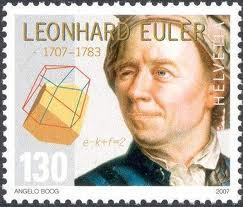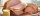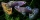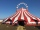# Euler problem

Someone buys a 180 tolars towels. If it was for the same money of 3 more towels, it would be 3 tolars cheaper each. How many were towels?

Correct result:

n =  12

#### Solution:

$180=c n \ \\ 180 = (c-3)(n+3) \ \\ n>0 \ \\ \ \\ n = 12 \ \\ c = 15$We would be very happy if you find an error in the example, spelling mistakes, or inaccuracies, and please send it to us. We thank you!Tips to related online calculators
Looking for help with calculating roots of a quadratic equation?
Do you have a system of equations and looking for calculator system of linear equations?

## Next similar math problems:

• A fishermanA fisherman buys carnivores to fish. He could buy either 6 larvae and 4 worms for $132 or 4 larvae and 7 worms per$ 127. What is the price of larvae and worms? Argue the answer.
• Hotel roomsIn the 45 rooms, there were 169 guests, some rooms were three-bedrooms and some five-bedrooms. How many rooms were?
• The dormitoryThe dormitory accommodates 150 pupils in 42 rooms, some of which are triple and some are quadruple. Determine how many rooms are triple and how many quadruples.
• CaseyCasey bought a 15.4 pound turkey and an 11.6 pound ham for thanksgiving and paid $38.51. Her friend Jane bought a 10.2 pound turkey and a 7.3 pound ham from the same store and paid$24.84. Find the cost per pound of turkey and the cost per pound of ham.
• AntennasIf you give me two antennas will be same. If you give me again your two antenna I have a 5× so many than you. How many antennas have both mans?
• Pears and orangesThere are 3 times as many pears as oranges. If a group of children receives 5 oranges each, there will be no oranges left over. If the same group of children receives 8 pears each, there will be 21 pears leftover. How many children and oranges are there?
• Money duoJulius and Mark have together 45 euros. Mark has 50% more money than Julius. Determine the amount of money that have Mark and Julius.
• Guppies for salePaul had a bowl of guppies for sale. Four customers were milling around the store. 1. Rod told paul - I'll take half the guppies in the bowl, plus had a guppy. 2. Heather said - I'll take half of what you have, plus half a guppy. The third customer, Nancy
• Nine booksNine books are to be bought by a student. Art books cost $6.00 each and biology books cost$6.50 each . If the total amount spent was $56.00, how many of each book was bought? • CircusOn the circus performance was 150 people. Men were 10 less than women and children 50 more than adults. How many children were in the circus? • Factory and divisionsThe factory consists of three auxiliary divisions total 2,406 employees. The second division has 76 employees less than 1st division and 3rd division has 212 employees more than the 2nd. How many employees has each division? • Linear systemSolve this linear system (two linear equations with two unknowns): x+y =36 19x+22y=720 • Equations - simpleSolve system of linear equations: x-2y=6 3x+2y=4 • TicketsTickets to the zoo cost$4 for children, $5 for teenagers and$6 for adults. In the high season, 1200 people come to the zoo every day. On a certain day, the total revenue at the zoo was \$5300. For every 3 teenagers, 8 children went to the zoo. How many t
• Three unknownsSolve the system of linear equations with three unknowns: A + B + C = 14 B - A - C = 4 2A - B + C = 0
• Three brothersThe three brothers have a total of 42 years. Jan is five years younger than Peter and Peter is 2 years younger than Michael. How many years has each of them?
• Two equationsSolve equations (use adding and subtracting of linear equations): -4x+11y=5 6x-11y=-5